# IPEC solver: Numerical simulation tool of polyion complex aggregation

IPEC solver is a windows program designed to analyse the stability of core-shell polyelectrolyte complexes formed by complexation of oppositely charged block co-polymers. The two-dimensional size distribution (number of anions and cations) of the complexes is calculated based on scaling model of block co-polymer aggregation and Poisson-Boltzmann theory for electrostatic interactions (VA Baulin, E. Trizac, Soft Matter, 8(25), 6755-6766 (2012)). Salt effects, charge distribution and distributions of small ions around the complexes are provided.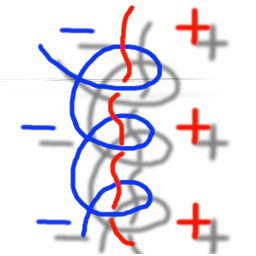This free software is designed to illustrate the physical mechanisms behind inter polyelectrolyte complexes self-assembly in salty media. It can suggest optimal structure of the aggregates in function of the structure of block copolymers and the conditions of aqueous solution. If you use this program, please site the article. If you are interested in a particular application of the Poisson-Boltzmann theory to a concrete experimental system involving complexation of oppositely charged block copolymers or charged micelles, please contact the authors.
• Theory and model:
Vladimir Baulin (Tarragona, Spain) Emmanuel Trizac (Paris, France)
• Design:
• Numerical implementation:
Beibei Huang (Tarragona, Spain)
• Graphical interface:
Vladimir Baulin (Tarragona, Spain) Beibei Huang (Tarragona, Spain)

Universitat Rovira i Virgili, Av. Paisos Catalans, 26, Tarragona 43007, Spain

Use contact details to give us a feedback or suggestion.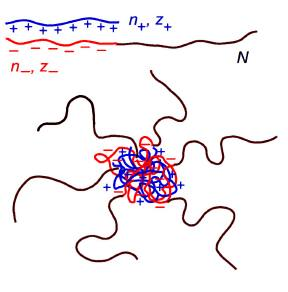## Screenshots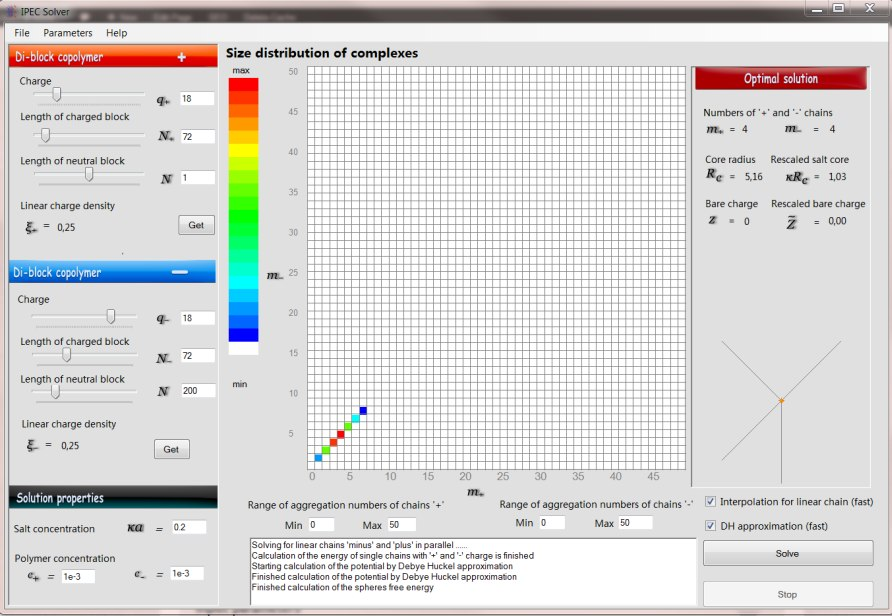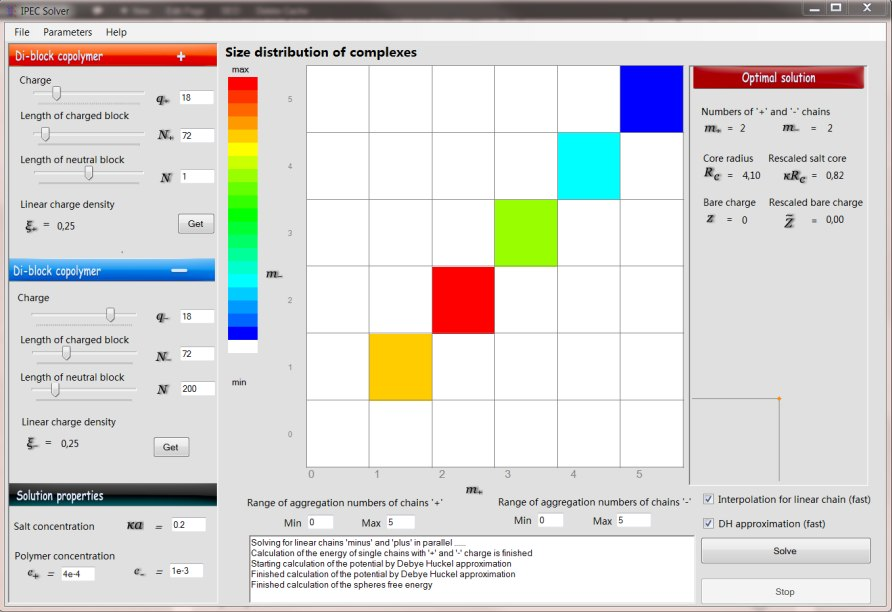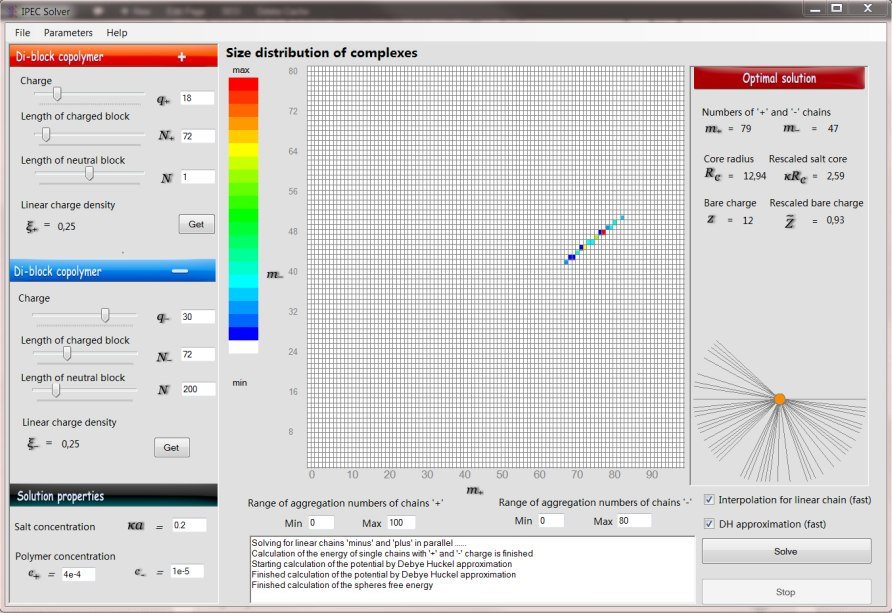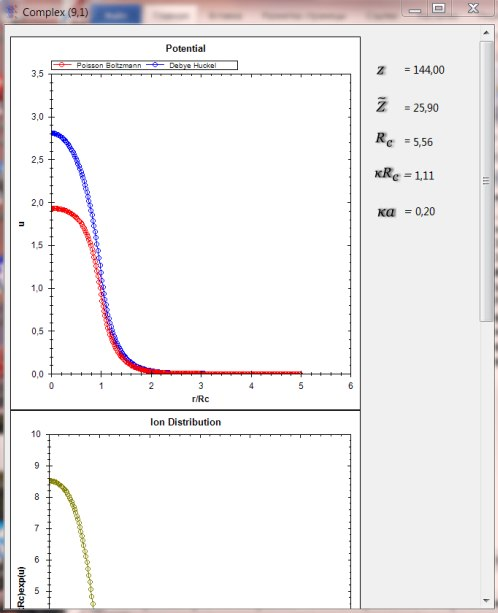## Input parameters

Toggle
Input parameters are divided in two groups:
##### The structure and geometry of the chains:

The charge of the block copolymer chains, the length of the charged blocks and the length of neutral blocks.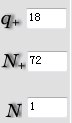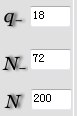##### Solution properties:

These parameters describe the bulk properties of the solution of block-copolymers.

ka is the salt concentration, while c+ and c- are the concentrations of unimers of positively and negatively charged polymers respectively.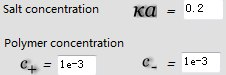The range of aggregation numbers of chains of both signs can be introduced in the windows below the grid.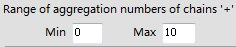Another way to introduce input parameters is to load directly from the file "parameter.in" containing all input parameters.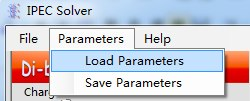When user choose "Save Parameters", IPEC will save all the input parameters into "parameter.in".

## Solving Equations

Toggle
User first choose two options of solution of equation.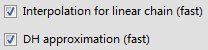First option "Interpolation for linear chain" allows to avoid expensive calculation of the Poisson-Boltzmann equations for linear chains and use interpolation of values stored in a databases "Chains_values.dat" and "Chains_values01.dat".. This should be good enough for approximate solution. However, if it is necessary to be sure in the solution, this option should be unchecked and the Poisson Boltzmann equations will be calculated. The second option, "DH approximation", allows to use Debye-Huckel approximation instead of the Poisson-Boltzmann equation (DH approximation unticked) for calculation of the electrostatic energy of the spherical complex. This option saves a lot of time because it uses analytical expressions instead of numerical solution. The performance of this approximation can be controlled by plotting the resulted electrostatic potential and comparison with the exact value obtained from numerical solution of the Poisson-Boltzmann equation. When IPEC solver starts calculations, user can stop it by click "stop", but when "DH approximation" is not ticked, it may cost some time to stop the PBE solution.

## Output distribution

Toggle
When IPEC solver finishes calculation, the properties of the complex of the maximum probability will be shown in the right column "Optimal solution" and the resulting size distribution of the complexes is shown on the grid with the colors from white to red indicating the probability of each complex containing a certain number of positively charged chains (bottom axis) and negatively charged chains (left axis).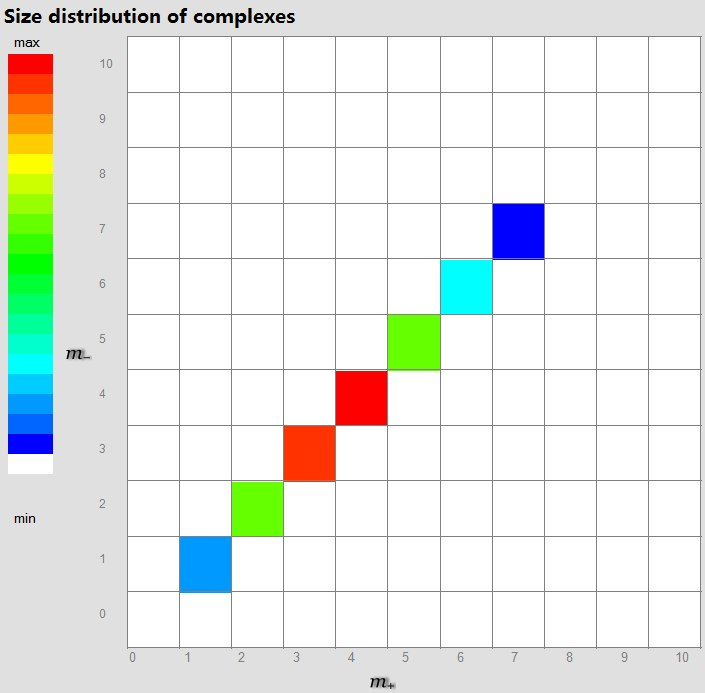User can save the distribution matrix choosing File->''Save Distribution".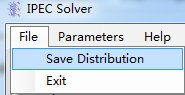## Electrostatic properties

Toggle
Once the size distribution is obtained, user can double click the grid with the corresponding coordinates.A new window will be open showing the electrostatic potential, ion distribution and charge density around the selected complex. The electrostatic potential is calculated in both, Debye-Huckel and Poisson-Boltzmann approximation. Right click opens the operation menu appear, where user can copy, print, save and zoom the plot.

## Get it!

• Win installation package
• Standard windows installation procedure
• Unpack zip-file
• Run setup.exe
• Size: 1,5MB